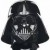# Problem Determining Statistical Differences

Six Sigma – iSixSigma Forums Old Forums General Problem Determining Statistical Differences

Viewing 2 posts - 1 through 2 (of 2 total)
• Author
Posts
• #40175

I have been given 4000 lines of data (continuous), each of which represents a averaged value of a given part number.  Each average was calculated using a different sample size.  The total of averaged values for each part number differs is size as well.

Are the sample sizes large enough to determine the statistical significance between part numbers (40+) and then between locations (40+).
Determine the power of each sample after the fact.
HELP.   This sounds intensive…non-normality, unequal variances, unequal sample sizes, data points consisting of averages, any suggetsions?

0
#123860Darth
Participant

You have more darn questions than anyone on this forum but at least you ask nicely.
Too many things going on to properly answer in this manner.  There are sample size issues, hypothesis testing using ANOVA or median tests depending on normality, power calculations.  I wouldn’t worry about differences in sample sizes.  The stats usually account for that.  If you are capable of using Minitab, this is not an onerous or burdensome task. In column one you would put the 4000 averages.  In column two you would put the 40 different part numbers matching the data and in column three put t he 40 locations.  To do your power you will have to determine the desired precision.  Put that in along with the sample sizes and up pops the Power.

0
Viewing 2 posts - 1 through 2 (of 2 total)

The forum ‘General’ is closed to new topics and replies.